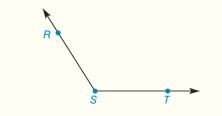Chapter 1.CT, Problem 18CT### Elementary Geometry for College St...

6th Edition
Daniel C. Alexander + 1 other
ISBN: 9781285195698

#### Solutions

Chapter
Section### Elementary Geometry for College St...

6th Edition
Daniel C. Alexander + 1 other
ISBN: 9781285195698
Textbook Problem
1 views

# Construct the angle bisector of obtuse angle R S T .To determine

To construct:

The bisector of given angle.

Explanation

Given:

The given angle is RST.

The given figure is,

Approach:

Consider the given figure,

Figure (1)

Use compass to make an arc that intersect sides of RST at point A and B as shown in figure (2).

### Still sussing out bartleby?

Check out a sample textbook solution.

See a sample solution

#### The Solution to Your Study Problems

Bartleby provides explanations to thousands of textbook problems written by our experts, many with advanced degrees!

Get Started

#### Find more solutions based on key concepts